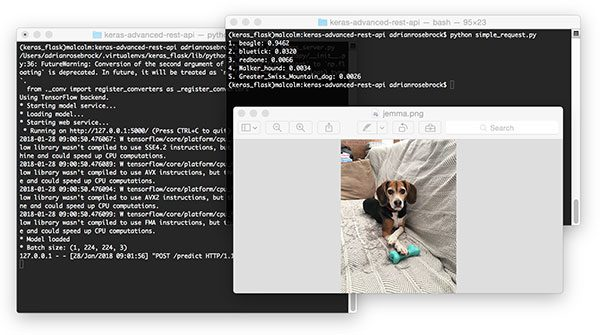搭建深度学习后台服务器

https://www.pyimagesearch.com/2018/01/29/scalable-keras-deep-learning-rest-api/#

1. KerasRedis(内存数据结构存储)
3. 消息队列和消息代理编程范例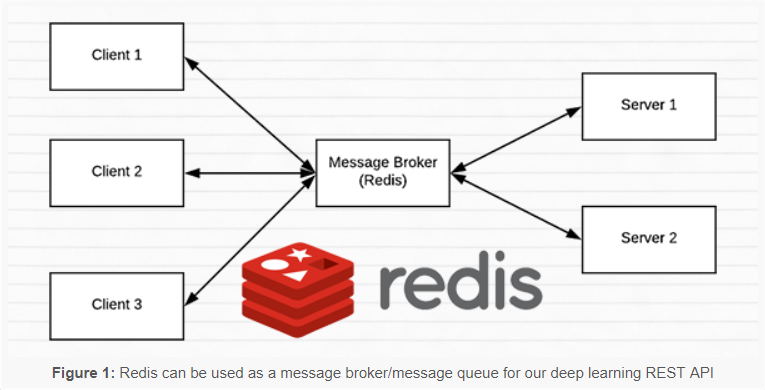图片1：Redis可以用作我们深度学习REST API的消息代理/消息队列

Redis是内存中的数据存储。它不同于简单的键/值存储(比如memcached)，因为它可以存储实际的数据结构。今天我们将使用Redis作为消息代理/消息队列。这包括:

1. 在我们的机器上运行Redis
2. 将数据(图像)按照队列的方式用Redis存储，并依次由我们的REST API处理
3. 为新批输入图像循环访问Redis
4. 对图像进行分类并将结果返回给客户端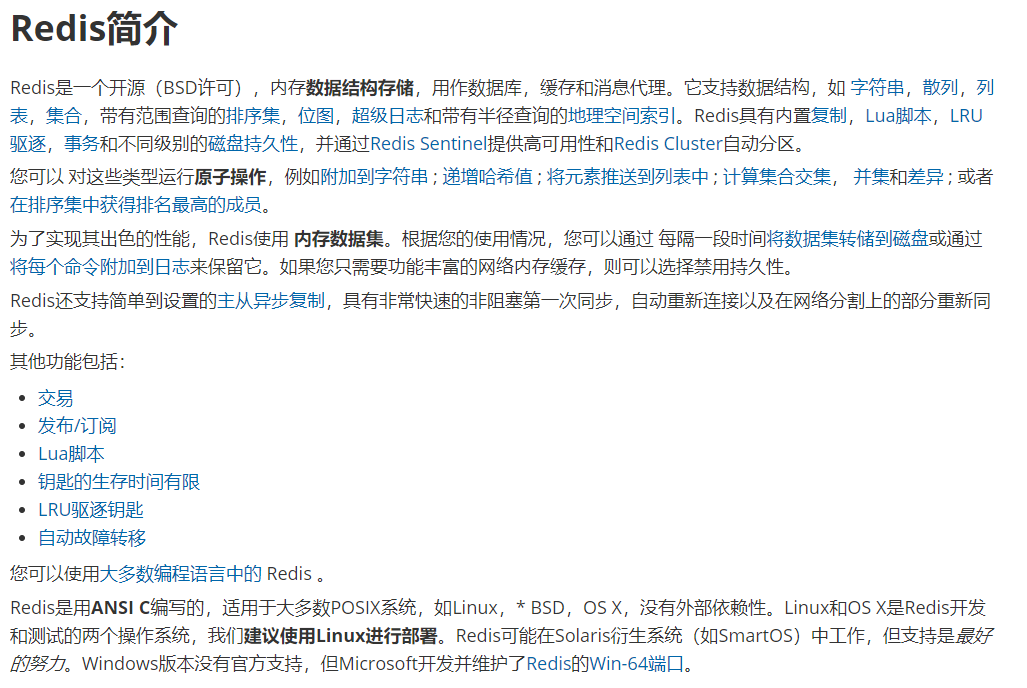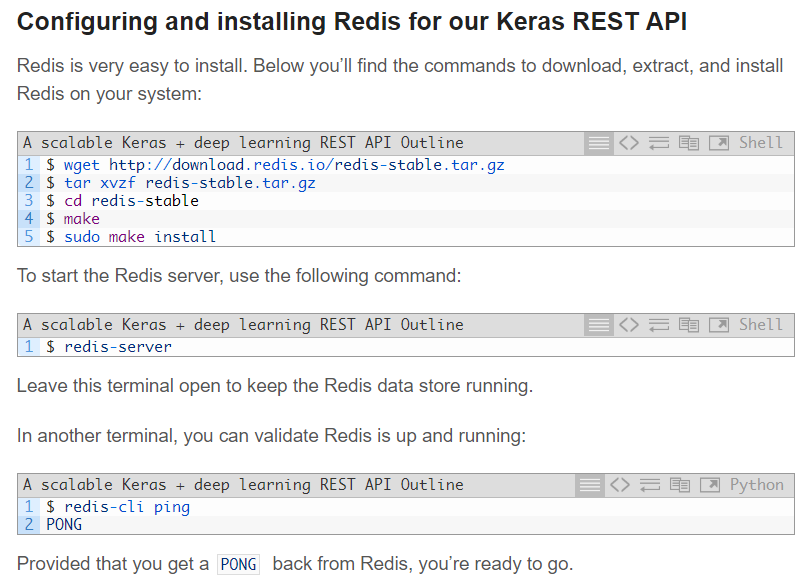conda install redis

resdis-server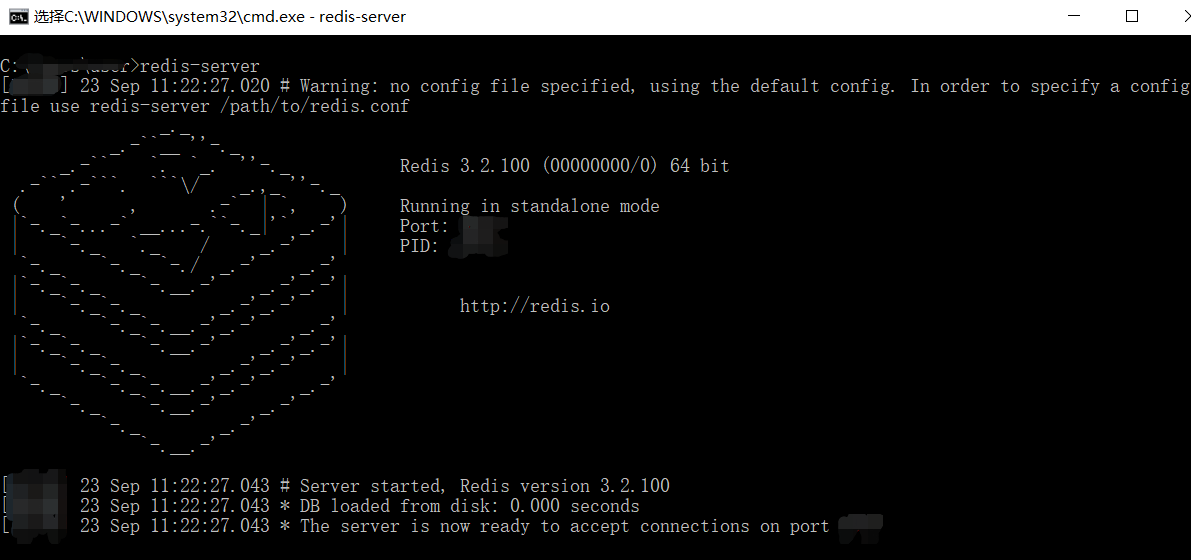redis-cli ping

$pip install numpy$ pip install scipy h5py
$pip install tensorflow # tensorflow-gpu for GPU machines$ pip install keras
$pip install flask gevent$ pip install imutils requests
$pip install redis$ pip install Pillow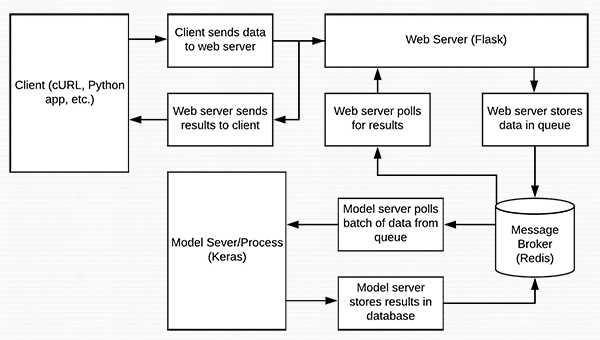# import the necessary packages
from keras.applications import ResNet50
from keras.preprocessing.image import img_to_array
from keras.applications import imagenet_utils
from PIL import Image
import numpy as np
import base64
import redis
import uuid
import time
import json
import sys
import io

# initialize constants used to control image spatial dimensions and
# data type
IMAGE_WIDTH = 224
IMAGE_HEIGHT = 224
IMAGE_CHANS = 3
IMAGE_DTYPE = "float32"

# initialize constants used for server queuing
IMAGE_QUEUE = "image_queue"
BATCH_SIZE = 32
SERVER_SLEEP = 0.25
CLIENT_SLEEP = 0.25

# initialize our Flask application, Redis server, and Keras model
db = redis.StrictRedis(host="localhost", port=6379, db=0)
model = None

def base64_encode_image(a):
# base64 encode the input NumPy array
return base64.b64encode(a).decode("utf-8")

def base64_decode_image(a, dtype, shape):
# if this is Python 3, we need the extra step of encoding the
# serialized NumPy string as a byte object
if sys.version_info.major == 3:
a = bytes(a, encoding="utf-8")

# convert the string to a NumPy array using the supplied data
# type and target shape
a = np.frombuffer(base64.decodestring(a), dtype=dtype)
a = a.reshape(shape)

# return the decoded image
return a

Redis将充当服务器上的临时数据存储。图像将通过诸如cURL、Python脚本甚至是移动应用程序等各种方法进入服务器，而且，图像只能每隔一段时间(几个小时或几天)或者以很高的速率(每秒几次)进入服务器。我们需要把图像放在某个地方，因为它们在被处理前排队。我们的Redis存储将作为临时存储。

base64_encode_image函数处理序列化。类似地，在通过模型传递图像之前，我们需要反序列化图像。这由base64_decode_image函数处理。

def prepare_image(image, target):
# if the image mode is not RGB, convert it
if image.mode != "RGB":
image = image.convert("RGB")

# resize the input image and preprocess it
image = image.resize(target)
image = img_to_array(image)
image = np.expand_dims(image, axis=0)
image = imagenet_utils.preprocess_input(image)

# return the processed image
return image

def classify_process():
# load the pre-trained Keras model (here we are using a model
# pre-trained on ImageNet and provided by Keras, but you can
# substitute in your own networks just as easily)
model = ResNet50(weights="imagenet")
print("* Model loaded")

classify_process函数将在它自己的线程中启动，我们将在下面的__main__中看到这一点。该函数将从Redis服务器轮询图像批次，对图像进行分类，并将结果返回给客户端。

	# continually poll for new images to classify
while True:
# attempt to grab a batch of images from the database, then
# initialize the image IDs and batch of images themselves
queue = db.lrange(IMAGE_QUEUE, 0, BATCH_SIZE - 1)  #第79行
imageIDs = []
batch = None

# loop over the queue
for q in queue:
# deserialize the object and obtain the input image
image = base64_decode_image(q["image"], IMAGE_DTYPE,
(1, IMAGE_HEIGHT, IMAGE_WIDTH, IMAGE_CHANS))

# check to see if the batch list is None
if batch is None:
batch = image

# otherwise, stack the data
else:
batch = np.vstack([batch, image])

# update the list of image IDs
imageIDs.append(q["id"])

		# check to see if we need to process the batch
if len(imageIDs) > 0:                     # 第102行
# classify the batch
print("* Batch size: {}".format(batch.shape))
preds = model.predict(batch)
results = imagenet_utils.decode_predictions(preds)

# loop over the image IDs and their corresponding set of
# results from our model
for (imageID, resultSet) in zip(imageIDs, results):
# initialize the list of output predictions
output = []

# loop over the results and add them to the list of
# output predictions
for (imagenetID, label, prob) in resultSet:
r = {"label": label, "probability": float(prob)}
output.append(r)

# store the output predictions in the database, using
# the image ID as the key so we can fetch the results
db.set(imageID, json.dumps(output))        #第122行

# remove the set of images from our queue
db.ltrim(IMAGE_QUEUE, len(imageIDs), -1)

# sleep for a small amount
time.sleep(SERVER_SLEEP)

@app.route("/predict", methods=["POST"])
def predict():
# initialize the data dictionary that will be returned from the
# view
data = {"success": False}

# ensure an image was properly uploaded to our endpoint
# read the image in PIL format and prepare it for
# classification
image = Image.open(io.BytesIO(image))
image = prepare_image(image, (IMAGE_WIDTH, IMAGE_HEIGHT))

# ensure our NumPy array is C-contiguous as well,
# otherwise we won't be able to serialize it
image = image.copy(order="C")

# generate an ID for the classification then add the
# classification ID + image to the queue
k = str(uuid.uuid4())
d = {"id": k, "image": base64_encode_image(image)}
db.rpush(IMAGE_QUEUE, json.dumps(d))

			# keep looping until our model server returns the output
# predictions
while True:
# attempt to grab the output predictions
output = db.get(k)

# check to see if our model has classified the input
# image
if output is not None:
# add the output predictions to our data
# dictionary so we can return it to the client
output = output.decode("utf-8")

# delete the result from the database and break
# from the polling loop
db.delete(k)
break

# sleep for a small amount to give the model a chance
# to classify the input image
time.sleep(CLIENT_SLEEP)

# indicate that the request was a success
data["success"] = True

# return the data dictionary as a JSON response
return flask.jsonify(data)

# if this is the main thread of execution first load the model and
# then start the server
if __name__ == "__main__":
# load the function used to classify input images in a *separate*
# thread than the one used for main classification
print("* Starting model service...")
t.daemon = True
t.start()

# start the web server
print("* Starting web service...")
app.run()

redis-server

python run_keras_server.py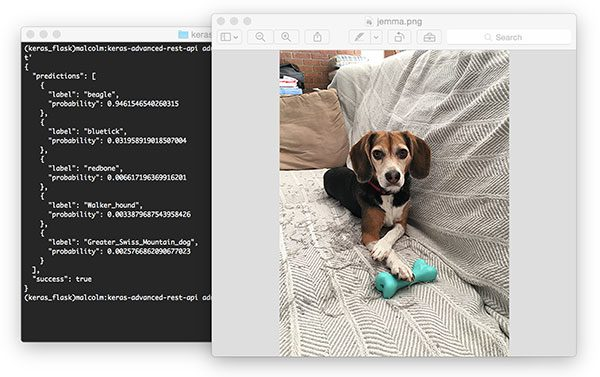curl -X POST -F image=@jemma.png 'http://localhost:5000/predict'

{
"predictions": [
{
"label": "beagle",
"probability": 0.9461546540260315
},
{
"label": "bluetick",
"probability": 0.031958919018507004
},
{
"label": "redbone",
"probability": 0.006617196369916201
},
{
"label": "Walker_hound",
"probability": 0.0033879687543958426
},
{
"label": "Greater_Swiss_Mountain_dog",
"probability": 0.0025766862090677023
}
],
"success": true
}

# import the necessary packages
import requests

# initialize the Keras REST API endpoint URL along with the input
# image path
KERAS_REST_API_URL = "http://localhost:5000/predict"
IMAGE_PATH = "jemma.png"

# load the input image and construct the payload for the request

# submit the request

# ensure the request was sucessful
if r["success"]:
# loop over the predictions and display them
for (i, result) in enumerate(r["predictions"]):
print("{}. {}: {:.4f}".format(i + 1, result["label"],
result["probability"]))

# otherwise, the request failed
else:
print("Request failed")

python simple_request.py